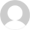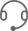•广告合作

### 新人法师farm入门

7.11编按:nga的图片上传真是ooxx啊,图片老挂,不过难得有其他网站转载了图片,使得我能把图片找回.以后再也不用nga的图片上传功能了><~

1.   插件(2.1下已无用)

zcc

Omincc

supermacro

2.   天赋

1-44级选择火法,火法的farm过程为,大火球,火球火球,nova(冰霜新星),火球循环,最后火冲或者魔杖结束

3.   装备选择

fm方面除了鞋子f个加速以外,其他fm都不是必须

4.   按键设置

1.1级寒冰箭 2.顶级寒冰箭 3.火冲 f.blink(闪现) 4.coc(冰锥) 5.魔杖 r.变羊 t.灼烧 g.冰箱 h.奥术飞弹 q.nova(冰霜新星) e.cs(反制)

F1.1级blz F2.烈焰风暴 F3.唤醒 F4.顶级blz F5.冰盾(鼠标滚轮向下) z.绷带 x.吃尸体(ud天赋) c.奥爆 v.法力护盾 alt+q.吃法力宝石 alt+w.缓落 alt+e.急冷(鼠标滚轮向上)

5.   宏(2.1下已无用)

coc

`/script local c,i,b,f=CastSpellByName;for i=1,16 do b=UnitBuff("player",i);if b and strfind(b,"ManaB") then f=1;break;end;end;if f or ((UnitMana("player")/UnitManaMax("player"))>0.1) then c("冰锥术");else c("冰锥术(等级 1)");end`

`/script local c,i,b,f=CastSpellByName;for i=1,16 do b=UnitBuff("player",i);if b and strfind(b,"ManaB") then f=1;break;end;end;if f or ((UnitMana("player")/UnitManaMax("player"))>0.4) then c("魔爆术");else c("魔爆术(等级 1)");end`

farm公主

`/script local c,_,d,i,b,f=CastSpellByName,GetActionCooldown(你气定动作条的编号);for i=1,16 do b=UnitBuff("player",i);if b and strfind(b,"antAr") then f=1;break;end;end;if f then c("炎爆术");elseif d>0 then c("火焰冲击");else c("气定神闲");end;`

`/script Iceblock();`

`function Iceblock()`

`   local c,i,b,f=CastSpellByName;s,d=GetActionCooldown(你冰箱的动作条编号);r=GetTime()-s;`

`   for i=1,16 do`

`      b=UnitBuff("player",i);`

`      if b and strsub(b,29)=="Frost" then f=1;`

`      break;`

`      end;`

`   end;`

`   if not f then`

`      if d>2 then c("急速冷却");else c("寒冰屏障");end;`

`   elseif ((d>2 and r>0.5) or IsAltKeyDown()) then c("寒冰屏障");`

`   end;`

`end`

`/script LazyMage();`

Code c:

`function LazyMage()`

`   local c,s,d,r,i,b,f=CastSpellByName;`

`   s,d=GetActionCooldown(你coc的动作条编号);`

`   r=d+s-GetTime();`

`   for i=1,16 do`

`      b=UnitBuff("player",i);`

`      if b and strfind(b,"ManaB") then f=1;break;`

`      end;`

`   end;`

`   if (d>2 and r>1.5) then`

`      if f or ((UnitMana("player")/UnitManaMax("player"))>0.4) then`

`         c("魔爆术");`

`      else`

`         c("魔爆术(等级 1)");`

`      end;`

`   else`

`      if f or ((UnitMana("player")/UnitManaMax("player"))>0.1) then`

`         c("冰锥术");`

`      else`

`         c("冰锥术(等级 1)");`

`      end;`

`   end;`

`end`

`/script ConjureManaStone();`

Code c:

`function ConjureManaStone()`

`   local x,j,b,s,l,f={"红宝石","黄水晶","翡翠","玛瑙"},1;`

`   while j<5 do`

`      f=nil;`

`      for b=0,4 do`

`         for s=1,18 do`

`            l=GetContainerItemLink(b,s);`

`            if l and strfind(l,"法力"..x[j]) then`

`               f=j;break;`

`            end;`

`         end;`

`         if f then break;end;`

`      end;`

`      if f then j=j+1;`

`      else CastSpellByName("制造魔法"..x[j]);break;`

`      end;`

`   end;`

`end;`

`/use 法力黄水晶`

`/use 法力翡翠`

`/use 法力玛瑙`

60级之前的路线,各个帖子都有各自的说法,我仅写出我的升级过程

1-44:任务升级

44-46:塔纳利斯南海海盗

46-52:内mld虫子和乌龟

52-58:西瘟疫2号农场

58-60:zg鳄鱼

44级出了新的blz,这时海盗在你眼里就是一堆一堆的经验,如果没有人打扰,这里是最好的升级地方,没有之一,随随便便就能到4w+/h.不过这个如果经常不存在,我身在pve服务器,还是人满为患.虽然不用担心有敌对阵营的追杀,但是只要多2个fs和你竞争aoe,效率就没了.

45级可以去zul aoe了

46级可以去mld了,这里确实是fs的天堂,6w/h,到了50+也有5w,一直可以a到52级

3批是左边一批,锅和右上一批,右边房后一批.另外,不要引到血色那帮人

58级可以去zg了,开始你可能会交点学费,但是等你熟练之后,估计就60了

58级去刷鳄鱼,我建议一开始尝试的时候2堆2堆的刷(这里可以用魔甲,不过在最初尝试的时候为安全可以用冰甲).这个时候你不用担心命中的问题,在元素精准的帮助下,你对鳄鱼(60级mob)的命中已能达到99%

1级：95%

2级：94%

3级:pve为83%,pvp为87%

4级:pve为72%,pvp为80%

5级:pve为61%,pvp为73%

1.   mld公主

2.   zg鳄鱼

60数据库|wowfan

70数据库|wowfan

80数据库|wowfan

• ##### 魔兽世界怀旧服数据库

怀旧服数据库|wowfan